Function Repository Resource:

# FindLongestPath

Find the longest path between two vertices in a directed acyclic graph

Contributed by: Charles Pooh
 ResourceFunction["FindLongestPath"][g,s,t] finds the longest path from source vertex s to target vertex t in the directed acyclic graph g. ResourceFunction["FindLongestPath"][{v→w,…},…] uses rules v→w to specify the graph g.

## Details and Options

ResourceFunction["FindLongestPath"][g,s,t] gives a path from s to t.
ResourceFunction["FindLongestPath"] can only be used for directed acyclic graphs (DAGs).
ResourceFunction["FindLongestPath"] uses the Bellman–Ford algorithm on a DAG with negative weights.

## Examples

### Basic Examples (2)

Find the longest path from the root of a directed acyclic graph (DAG) to a leaf:

 In:=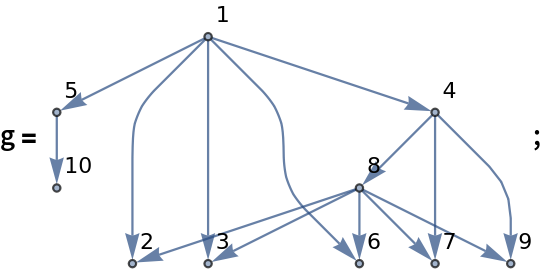In:=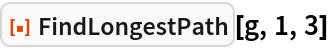Out=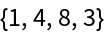Highlight the longest path:

 In:=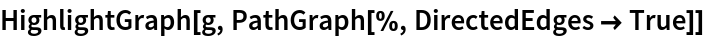Out=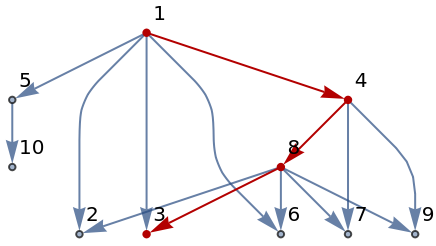### Scope (2)

FindLongestPath works with directed acyclic graphs:

 In:=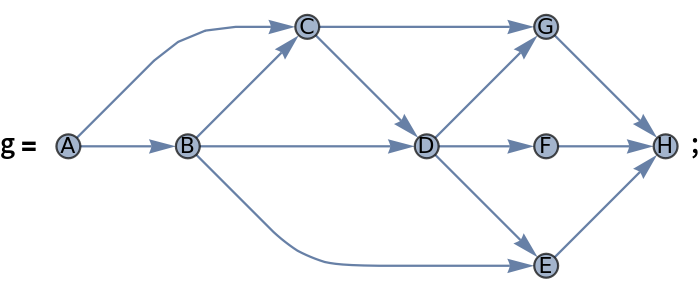In:=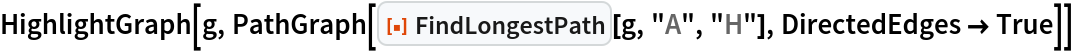Out=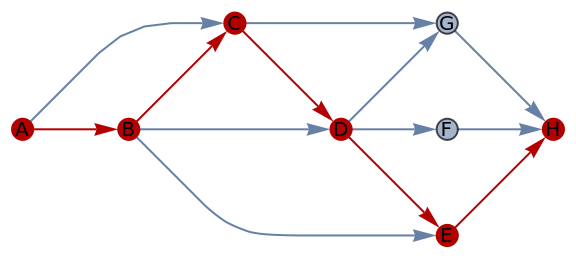Use rules to specify the graph:

 In:=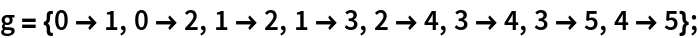In:=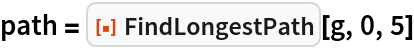Out=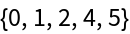In:=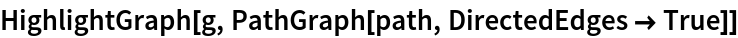Out=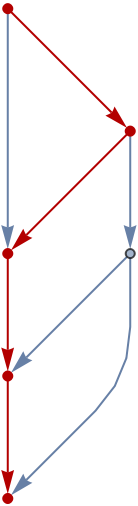### Possible Issues (1)

FindLongestPath is left unevaluated if the input graph is not a DAG:

 In:=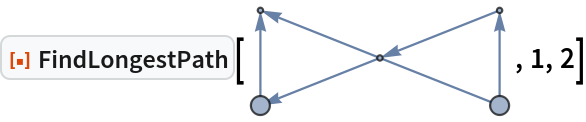Out=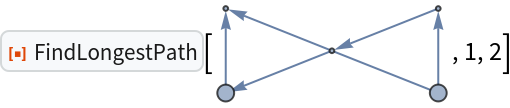## Version History

• 1.0.0 – 18 December 2020